Courses

# RD Sharma Solutions - Ex-25.1, Data Handling IV Probability, Class 7, Math Class 7 Notes | EduRev

## Class 7 : RD Sharma Solutions - Ex-25.1, Data Handling IV Probability, Class 7, Math Class 7 Notes | EduRev

The document RD Sharma Solutions - Ex-25.1, Data Handling IV Probability, Class 7, Math Class 7 Notes | EduRev is a part of the Class 7 Course RD Sharma Solutions for Class 7 Mathematics.
All you need of Class 7 at this link: Class 7

#### Question 1:

A coin is tossed 1000 times with the following frequencies:

When a coin is tossed at random, what is the probability of getting (i) a head? (ii) a tail?

Total number of times a coin is tossed = 1000
Number of times a head comes up = 445
Number of times a tail comes up = 555

(i) Probability of getting a head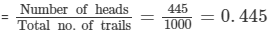(ii) Probability of getting a tail =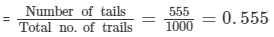#### Question 2:

A die is thrown 100 times and outcomes are noted as given below:

 Outcome: 1 2 3 4 5 6 Frequency: 21 9 14 23 18 15

If a die is thrown at random, find the probability of getting a/an.
(i) 3
(ii) 5
(iii) 4
(iv) Even number
(v) Odd number
(vi) Number less than 3.

Total number of trials = 100

Number of times "1" comes up = 21
Number of times "2" comes up = 9
Number of times "3" comes up = 14
Number of times "4" comes up = 23
Number of times "5" comes up = 18
Number of times "6" comes up = 15

(i) Probability of getting 3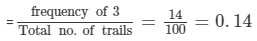(ii) Probability of getting 5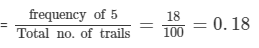(iii) Probability of getting 4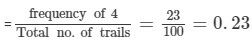(iv) Frequency of getting an even no. = Frequency of 2 + Frequency of 4 + Frequency of 6 = 9+ 23 + 15 = 47

Probability of getting an even no.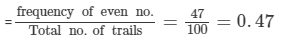(v) Frequency of getting an  odd no. = Frequency of 1 + Frequency of 3 + Frequency of 5 = 21+ 14 + 18 = 53

Probability of getting an odd no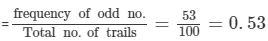(vi) Frequency of getting a no. less than 3 = Frequency of 1 + Frequency of 2= 21 + 9 = 30

Probability of getting a no. less than 3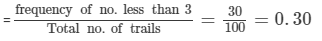#### Question 3:

A box contains two pair of socks of two colours (black and white). I have picked out a white sock. I pick out one more with my eyes closed. What is the probability that I will make a pair?

No. of socks in the box = 4
Let B and W denote black and white socks respectively.
Then we have:
S = {B,B,W,W}

If a white sock is picked out, then the total no. of socks left in the box = 3
No. of white socks left = 2-1 =1

Probability of getting a white sock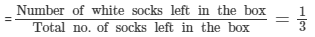#### Question 4:

Two coins are tossed simultaneously 500 times and the outcomes are noted as given below:

If same pair of coins is tossed at random, find the probability of getting (i) Two heads (ii) One head (iii) No head.

Number of trials = 500
Number of outcomes of two heads (HH) =  105
Number of outcomes of one head (HT or TH) =  275
Number of outcomes of no head (TT) =  120

(i) Probability of getting two heads(ii) Probability of getting one head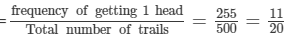(iii) Probability of getting no head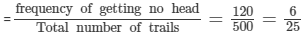Offer running on EduRev: Apply code STAYHOME200 to get INR 200 off on our premium plan EduRev Infinity!

97 docs

,

,

,

,

,

,

,

,

,

,

,

,

,

,

,

,

,

,

,

,

,

,

,

,

,

,

,

,

,

,

;